# Texas Go Math Grade 3 Lesson 6.2 Answer Key Skip Count on a Number Line

Refer to our Texas Go Math Grade 3 Answer Key Pdf to score good marks in the exams. Test yourself by practicing the problems from Texas Go Math Grade 3 Lesson 6.2 Answer Key Skip Count on a Number Line.

## Texas Go Math Grade 3 Lesson 6.2 Answer Key Skip Count on a Number Line

Unlock the Problem

Caleb wants to make 3 balls of yarn for his cat to play with. He uses 6 feet of yarn to make each ball. How many feet of yarn does Caleb need in all?

How many equal groups of yarn will Caleb make?
3 equal groups
Explanation:
Caleb wants to make 3 balls of yarn for his cat to play with.
He uses 6 feet of yarn to make each ball.
Total feet of yarn Caleb need in all
3 × 6 = 18 feet

How many feet of yarn will be in each group?
6 feet
Explanation:
The above information in the problem tells that each group needs 6 feet of yarn.

What do you need to find?
How many feet of yarn does Caleb need in all?

Use a number line to count equal groups.

How many feet of yarn does Caleb need for each ball? ___________
How many equal lengths of yarn does he need? ___________
Begin at 0. Skip count by 6s by drawing jumps on the number line.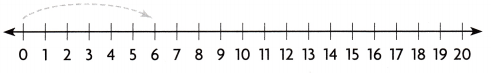How many jumps did you make? ___________
How long is each jump? ___________
Multiply. 3 × 6 = ___________
So, Caleb needs ___________ feet of yarn in all.
How many feet of yarn does Caleb need for each ball?
6 feet
How many equal lengths of yarn does he need?
He need three equal lengths of yarn.
Explanation:
The information given in the above problem,
tells about the number of feet and equal lengths of yarn.
Begin at 0. Skip count by 6s by drawing jumps on the number line.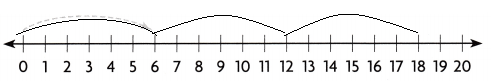How many jumps did you make? ____3_______
How long is each jump? _____6______
Multiply. 3 × 6 = _____18______
So, Caleb needs _____18______ feet of yarn in all.

Why did you jump by 6s on the number line?
Its a group of 6 feet
Explanation:
The above number line shows the skip counting of 6s,
So, each group has 6 feet.

Math Talk
Mathematical Processes

What if Caleb made 4 balls of yarn with 5 feet of yarn in each ball? What would you do differently to find the total number of feet of yarn?
4 x 5 = 20
Explanation:
Caleb made 4 balls of yarn with 5 feet of yarn in each ball.
The total number of feet of yarn needed
4 x 5 = 20
Share and Show

Question 1.
Skip count by drawing jumps on the number line, Find how many in 5 jumps of 4. Then write the product.
Think: 1 jump of 4 shows 1 group of 4.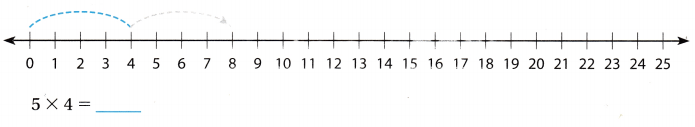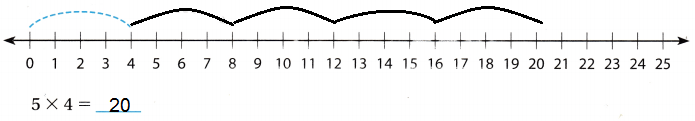Explanation:
The number line shows the skip counting of 4s in a group.
Total 5 groups, each group has 4s.
5 x 4 = 20

Draw jumps on the number line to show equal groups. Find the product.

Question 2.
3 groups of 8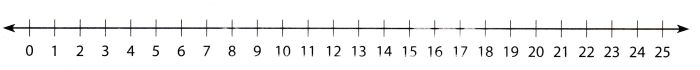3 × 8 = ___________3 x 8 = 24
Explanation:
The number line shows the skip counting of 8s in a group.
Total 3 groups, each group has 8s.
3 x 8 = 24

Question 3.
8 groups of 38 × 3 = _____________8 x 3 = 24
Explanation:
The number line shows the skip counting of 3s in a group.
Total 8 groups, each group has 3s.
8 x 3 = 24

Write the multiplication sentence the number line shows.

Question 4.________ × _________ = ___________
2 × 9 = 18
Explanation:
A number line can be defined as a straight line with numbers placed at equal intervals or segments along its length.
The number line shows the skip counting of 9s in a group.
Total 2 groups, each group has 9s.
2 x 9 = 18

Math Talk
Mathematical Processes

How do equal jumps on the number line show equal groups?
A number line can be defined as a straight line with numbers placed at equal intervals or segments along its length.
Explanation:
For example,
1 x 1
2 x 2
3 x 3
4 x 4…… and so on.
Number of jumps and number of groups must be the same,
Such jumps can be of  equal jumps on the number line show equal groups.

Problem Solving

Question 5.
Multi-Step Erin displays her toy cat collection ‘ on 3 shelves. She puts 8 cats on each shelf. If she collects 3 more cats, how many cats will she have?
27 cats
Explanation:
Erin displays her toy cat collection ‘ on 3 shelves.
She puts 8 cats on each shelf.
If she collects 3 more cats,
then total number of cats she have
3 x 8 =24
24 + 3 = 27

Question 6.
H.O.T. Write two multiplication sentences that have a product of 12. Draw jumps on the number line to show the multiplication.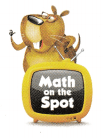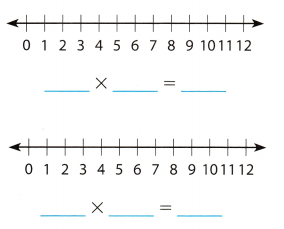3 x 4 =12
4 x 3 = 12Explanation:
A number line can be defined as a straight line with numbers placed at equal intervals or segments along its length.
Number of jumps and number of groups must be the same,
Such jumps can be of  equal jumps on the number line show equal groups.

Question 7.
Evaluate Sam, Kyra, Tia, and Abigail each have 10 pennies. How many pennies do they have in all?
40 pennies
Explanation:
Sam, Kyra, Tia, and Abigail each have 10 pennies.
Total pennies they have in all
4 x 10 =40

Question 8.
H.O.T. Pose a Problem Write a problem that can be solved by finding 8 groups of 5. Write a multiplication sentence to solve the problem. Then solve.
3rd Grade students formed eight groups and collected 5 books each group, how many books collected altogether?
Explanation:
each group collects 5 books
Total books collected altogether
8 x 5 = 40

Fill in the bubble for the correct answer choice.

Question 9.
Representations Eddie bought snacks for a picnic. He has 3 bags of snacks. Each bag has 4 snacks. How many snacks does Eddie have in all?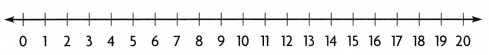(A) 7
(B) 16
(C) 12
(D) 8
Option (C)
Explanation :
Eddie has 3 bags of snacks.
Each bag has 4 snacks.
Total snacks Eddie have in all
4 x 3 = 12

Question 10.
Representations A cook has 3 boxes of eggs. Each box holds 6 eggs. Which number sentence can be used to find the number of eggs the cook has?(A) 3 + 6 = 9
(B) 3 + 3 = 6
(C) 3 × 3 = 9
(D) 3 × 6 = 18
Option (D)
Explanation:
A cook has 3 boxes of eggs.
Each box holds 6 eggs.
Number of eggs the cook has
3 x 6 =18

Question 11.
Multi-Step Ashley digs 7 holes. She puts 2 seeds in each hole. She has 3 seeds left over. How many seeds are there in all?(A) 12
(B) 23
(C) 17
(D) 14
Option (C)
Explanation:
Ashley digs 7 holes.
She puts 2 seeds in each hole.
She has 3 seeds left over.
Total seeds in all
7 x 2 =14
14  + 3 = 17 seeds

Texas Test Prep

Question 12.
Brooke needs 4 lengths of string, each 2 inches long. How much string does she need altogether?
(A) 6 inches
(B) 4 inches
(C) 2 inches
(D) 8 inches
Option (D)
Explanation:
Brooke needs 4 lengths of string,
each 2 inches long.
Total string she need altogether
4 x 2 =8 inches

### Texas Go Math Grade 3 Lesson 6.2 Homework and Practice Answer Key

Draw jumps on the number line to show equal groups. Find the product.

Question 1.
4 groups of 64 × 6 = ___________4 x 6 = 24
Explanation:
A number line can be defined as a straight line with numbers placed at equal intervals or segments along its length.
Number of jumps and number of groups must be the same,
Such jumps can be of  equal jumps on the number line show equal groups.

Write the multiplication sentence the number line shows.
In a shop there are 6 eggs in one box, Kyle bought 4 boxes. How many eggs he bought in all?
4 x 6 = 24 eggs

Question 2.________ × _________ = __________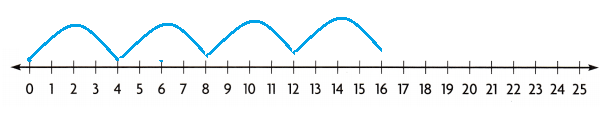4 x 4 =165 x 5 =25
Explanation:
A number line can be defined as a straight line with numbers placed at equal intervals or segments along its length.
Number of jumps and number of groups must be the same,
Such jumps can be of  equal jumps on the number line show equal groups.

Question 3.
Write two multiplication sentences that have a product of 16. Draw jumps on the number line to show the multiplication.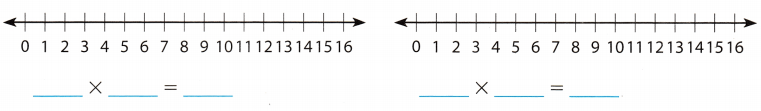Explanation:
A number line can be defined as a straight line with numbers placed at equal intervals or segments along its length.
Number of jumps and number of groups must be the same,
Such jumps can be of  equal jumps on the number line show equal groups.

Problem Solving

Question 4.
Hannah, Steven, and Nicole each have 9 books. How many books do they have in all?
27 books
Explanation:
Hannah, Steven, and Nicole each have 9 books.
Total books they have in all
3 x 9 = 27

Question 5.
Kyle has 6 crayons in 3 boxes. If he adds another crayon to each box, how many crayons will Kyle have?
18 crayons
Explanation:
Kyle has 6 crayons in 3 boxes.
If he adds another crayon to each box,
Total crayons Kyle have in altogether
6 x 3 = 18

Lesson Check

Question 6.
In Mr. Gupta’s classroom, there are 4 rows of desks. Each row has 6 desks. How many desks are in Mr. Gupta’s classroom?(A) 10
(B) 18
(C) 24
(D) 21
Option (C)
Explanation:
In Mr. Gupta’s classroom, there are 4 rows of desks.
Each row has 6 desks.
Number of desks in Mr. Gupta’s classroom
4 x 6 = 24

Question 7.
Matt wants to make 5 puppets. He needs 3 buttons for each puppet. Which number sentence can Matt use to find the number of buttons he needs?(A) 5 + 3 = 8
(B) 5 × 5 = 25
(C) 3 × 3 = 9
(D) 5 × 3 = 15
Option (D)
Explanation:
Matt wants to make 5 puppets.
He needs 3 buttons for each puppet.
Number of buttons he needs
5 x 3 = 15

Question 8.
Ichiro builds 3 birdhouses. He uses 6 pieces of wood for each birdhouse. How many pieces of wood does Ichiro use?(A) 9
(B) 18
(C) 15
(D) 24
Option (B)
Explanation:
Ichiro builds 3 birdhouses.
He uses 6 pieces of wood for each birdhouse.
Total pieces of wood Ichiro use
3 x 6 = 18

Question 9.
Multi-Step A band marches in rows of 5. Each row has 6 people. There are 4 people who carry flags. How many people are in the marching band?
(A) 15
(B) 24
(C) 34
(D) 30
Option (C)
Explanation:
A band marches in rows of 5.
Each row has 6 people.
There are 4 people who carry flags.
Number of people in the marching band
5 x 6  + 4
30 + 4 = 34

Question 10.
Multi-Step Carla puts 8 pictures on each page of a photo album. She fills 3 pages. She has 5 pictures left. How many pictures does she have?
(A) 24
(B) 15
(C) 15
(D) 29